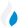•Trade Pi
•Trade
•Exchange
•Trade Pi
•Trade
•Exchange
\$1.12T
Total marketcap
\$66.05B
Total volume
39.64%
BTC dominance
•Trade Pi
•Trade
•Exchange

# matic to JPY Exchange Rate - 1 Polygon in JPY

162.026
• BTC 0.000054
• ETH 0.0008
Vol [24h]
\$860,024.07

## matic to JPY converter

Exchange Pair Price 24h volume
Bitbank MATIC/JPY \$1.22 \$852.01K

## MATIC/JPY Exchange Rate Overview

Name Ticker Price % 24h 24h high 24h low 24h volume
Polygon matic \$1.22 0.0665% \$1.24 \$1.19 \$487.44M

Selling 1 Polygon matic you get 162.026 JPY.

Polygon Dec 27, 2021 had the highest price, at that time trading at its all-time high of \$2.92.

407 days have passed since then, and now the price is 42.52% of the maximum.

Based on the table data, the MATIC vs JPY exchange volume is \$860,024.07.

Using the calculator/converter on this page, you can make the necessary calculations with a pair of Polygon to JPY.

## Q&A

### What is the current MATIC to JPY exchange rate?

Right now, the MATIC/JPY exchange rate is 162.026.

### What has been the Polygon to JPY trading volume in the last 24 hours?

Relying on the table data, the Polygon to JPY exchange volume is \$860,024.07.

### How can I calculate the amount of JPY? / How do I convert my Polygon to JPY?

You can calculate/convert JPY from MATIC to JPY converter. Also, you can select other currencies from the drop-down list.

## MATIC to JPY Сonversion Table

MATIC JPY
0.4 MATIC = 64.8 JPY
0.8 MATIC = 129.6 JPY
2 MATIC = 324.05 JPY
2 MATIC = 324.05 JPY
4 MATIC = 648.1 JPY
8 MATIC = 1,296.2 JPY
20 MATIC = 3,240.5 JPY
40 MATIC = 6,481.04 JPY
90 MATIC = 14,582.3 JPY
900 MATIC = 145,823.4 JPY
9000 MATIC = 1,458,234 JPY
90000 MATIC = 14,582,340 JPY
900000 MATIC = 145,823,400 JPY